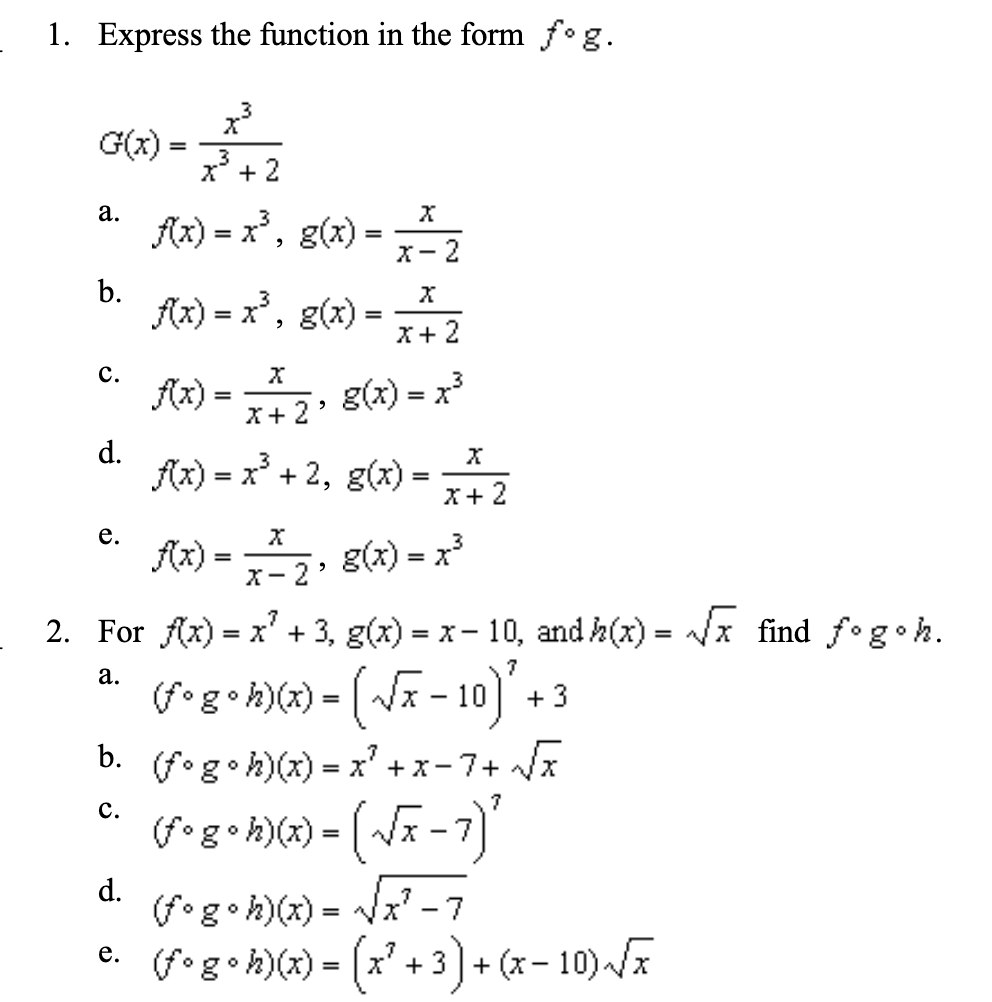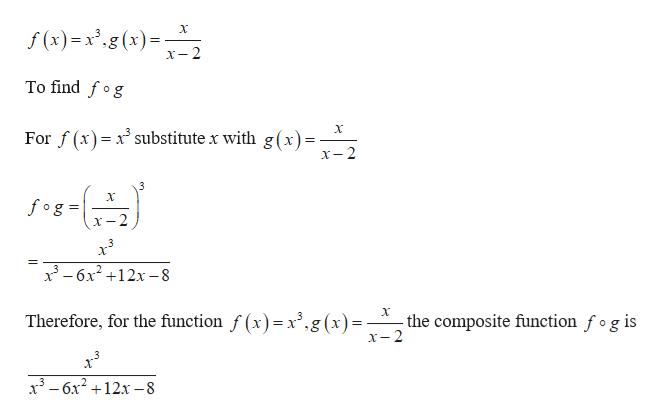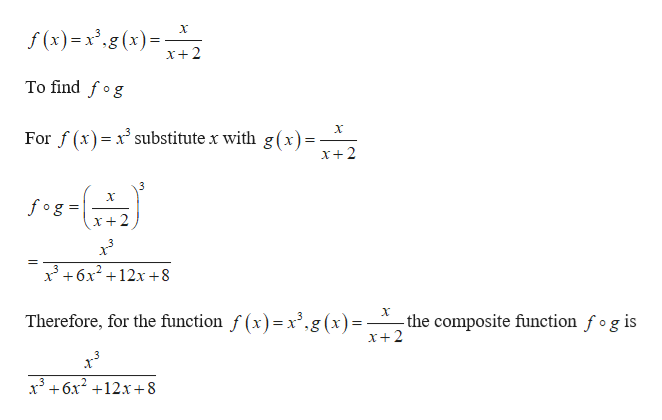# Express the function in the form f°g1.3G(x)32а.f)x, g)-2bfx)x, g)2с.g(x) xfx)2dfix)x2, g)е.fx)g(x) x322. For fx) x' +3, g(x) x-10, and A(z)x find fogoh=а.g -103b.(fog h)() xс.(fg.h))= -7)d(fg h))-(.3). -10)-x -7=+ (x-3

Question
10 viewshelp_outlineImage TranscriptioncloseExpress the function in the form f°g 1. 3 G(x) 3 2 а. f)x, g) -2 b fx)x, g) 2 с. g(x) x fx) 2 d fix)x2, g) е. fx) g(x) x3 2 2. For fx) x' +3, g(x) x-10, and A(z) x find fogoh = а. g -10 3 b. (fog h)() x с. (fg.h))= -7) d (fg h)) -(.3). -10)- x -7 = + (x- 3 fullscreen
check_circle

Step 1

“Since you have asked multiple questions with multiple sub-parts, we will solve the first three sub-parts of the first question for you. If you want any specific question with specific sub-parts to be solved then please specify the question number or post only that question.”

a)

Consider the given functionhelp_outlineImage Transcriptionclosef(x)=x",g(s)= 2 x-2 To find fog For f(x) xsubstitute x with g(x)- X-2 3 fog x-2 3-6x212x-8 Therefore, for the function f (x)= x',g (x)- the composite function fog is x-2 3-6x212x-8 fullscreen
Step 2

b)

Consider the given...help_outlineImage Transcriptionclosef (x)=.g(x) X+2 To find fog For f(x) xsubstitute x with g(x)- x+2 3 fog x+2 36x212x+8 X Therefore, for the function f (x)= x',g(x)- the composite function fog is x+2 x3+6x212x+8 fullscreen

### Want to see the full answer?

See Solution

#### Want to see this answer and more?

Solutions are written by subject experts who are available 24/7. Questions are typically answered within 1 hour.*

See Solution
*Response times may vary by subject and question.
Tagged in

### Functions